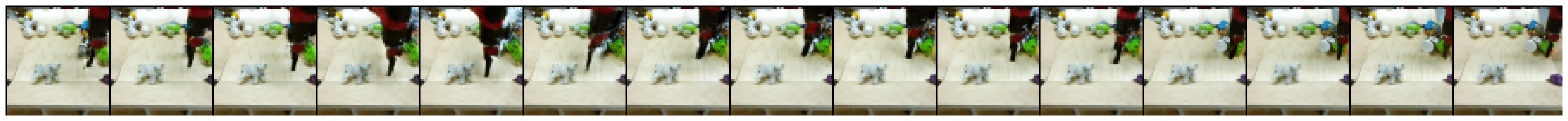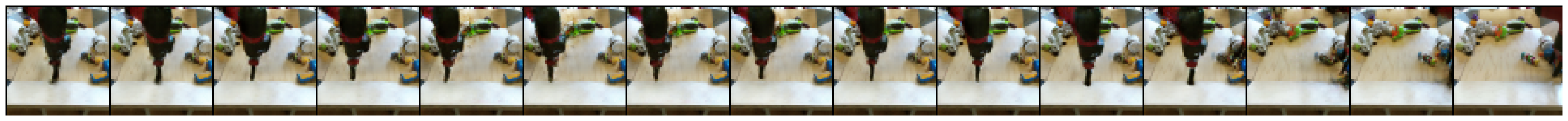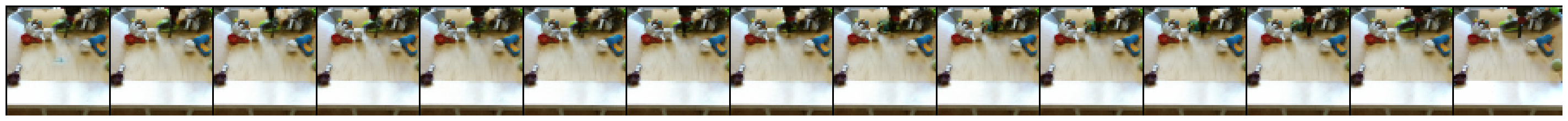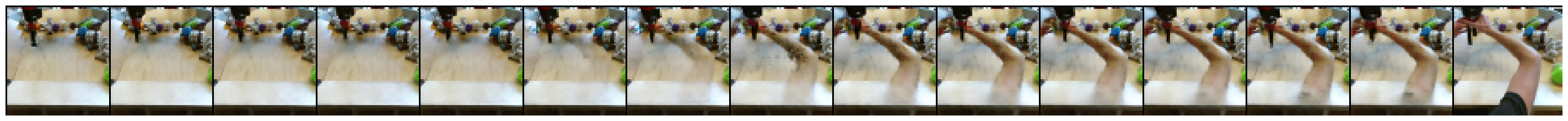This page was translated by the Cloud Translation API.

# 3 ডি কনভোলিউশনগুলি ব্যবহার করে ভিডিও অন্তর্নির্মিত

ইউপনেগ লি, ডোমিনিক রোবেলক, এবং মার্কো তাগলিয়াসাচি। এখানে থেকে সেখানে: সরাসরি 3 ডি কনভোলিউশনগুলি ব্যবহার করে ভিডিও অন্তর্নিহিতকরণ, 2019।

বর্তমান হাব বৈশিষ্ট্য:

• বিএআরআইআর রোবটকে পুশিং ভিডিও এবং কেটিএইচ অ্যাকশন ভিডিও ডেটাসেটের মডেল রয়েছে (যদিও এই কোলাবটি কেবল বিএআর ব্যবহার করে)
• হাবের মধ্যে ইতিমধ্যে বিয়ার ডেটাসেট উপলব্ধ। তবে, কেটিএইচ ভিডিওগুলি ব্যবহারকারীরা তাদের সরবরাহ করতে হবে।
• আপাতত কেবল মূল্যায়ন (ভিডিও প্রজন্ম)
• ব্যাচের আকার এবং ফ্রেমের আকার হার্ড-কোডড

## সেটআপ

যেহেতু tfds.load('bair_robot_pushing_small', split='test') একটি 30 গিগাবাইট সংরক্ষণাগারটি ডাউনলোড করবে যাতে প্রশিক্ষণের ডেটা রয়েছে, তাই আমরা একটি পৃথক সংরক্ষণাগার ডাউনলোড করি যেখানে কেবল 190MB পরীক্ষার ডেটা রয়েছে। ব্যবহৃত ডেটাসেটটি এই কাগজটি দ্বারা প্রকাশিত হয়েছে এবং ক্রিয়েটিভ কমন্স বাই ৪.০ হিসাবে লাইসেন্স পেয়েছে।

import tensorflow as tf

import matplotlib.pyplot as plt
import numpy as np
import seaborn as sns
import tensorflow_hub as hub
import tensorflow_datasets as tfds

from tensorflow_datasets.core import SplitGenerator
from tensorflow_datasets.video.bair_robot_pushing import BairRobotPushingSmall

import tempfile
import pathlib

TEST_DIR = pathlib.Path(tempfile.mkdtemp()) / "bair_robot_pushing_small/softmotion30_44k/test/"

# Download the test split to $TEST_DIR !mkdir -p$TEST_DIR

2020-06-12 12:14:36 URL:https://storage.googleapis.com/download.tensorflow.org/data/bair_test_traj_0_to_255.tfrecords [189852160/189852160] -> "/tmp/tmp67j3lrjq/bair_robot_pushing_small/softmotion30_44k/test/traj_0_to_255.tfrecords" 


# Since the dataset builder expects the train and test split to be downloaded,
# patch it so it only expects the test data to be available
builder = BairRobotPushingSmall()
test_generator = SplitGenerator(name='test', gen_kwargs={"filedir": str(TEST_DIR)})
builder._split_generators = lambda _: [test_generator]

Downloading and preparing dataset bair_robot_pushing_small/2.0.0 (download: 30.06 GiB, generated: Unknown size, total: 30.06 GiB) to /home/kbuilder/tensorflow_datasets/bair_robot_pushing_small/2.0.0...

HBox(children=(FloatProgress(value=1.0, bar_style='info', max=1.0), HTML(value='')))
WARNING:tensorflow:From /home/kbuilder/.local/lib/python3.6/site-packages/tensorflow_datasets/video/bair_robot_pushing.py:106: tf_record_iterator (from tensorflow.python.lib.io.tf_record) is deprecated and will be removed in a future version.
Instructions for updating:
Use eager execution and:
tf.data.TFRecordDataset(path)

Warning:tensorflow:From /home/kbuilder/.local/lib/python3.6/site-packages/tensorflow_datasets/video/bair_robot_pushing.py:106: tf_record_iterator (from tensorflow.python.lib.io.tf_record) is deprecated and will be removed in a future version.
Instructions for updating:
Use eager execution and:
tf.data.TFRecordDataset(path)

Shuffling and writing examples to /home/kbuilder/tensorflow_datasets/bair_robot_pushing_small/2.0.0.incompleteDMJA7H/bair_robot_pushing_small-test.tfrecord

HBox(children=(FloatProgress(value=0.0, max=256.0), HTML(value='')))
HBox(children=(FloatProgress(value=0.0, description='Computing statistics...', max=1.0, style=ProgressStyle(de…
WARNING:tensorflow:From /home/kbuilder/.local/lib/python3.6/site-packages/tensorflow_datasets/core/features/feature.py:332: calling map_fn_v2 (from tensorflow.python.ops.map_fn) with back_prop=False is deprecated and will be removed in a future version.
Instructions for updating:
results = tf.map_fn(fn, elems, back_prop=False)
Use:

Warning:tensorflow:From /home/kbuilder/.local/lib/python3.6/site-packages/tensorflow_datasets/core/features/feature.py:332: calling map_fn_v2 (from tensorflow.python.ops.map_fn) with back_prop=False is deprecated and will be removed in a future version.
Instructions for updating:
results = tf.map_fn(fn, elems, back_prop=False)
Use:

HBox(children=(FloatProgress(value=1.0, bar_style='info', max=1.0), HTML(value='')))

Dataset bair_robot_pushing_small downloaded and prepared to /home/kbuilder/tensorflow_datasets/bair_robot_pushing_small/2.0.0. Subsequent calls will reuse this data.



## BAIR: নমপি অ্যারে ইনপুটগুলির উপর ভিত্তি করে ডেমো

# @title Load some example data (BAIR).
batch_size = 16

ds = builder.as_dataset(split="test")
test_videos = ds.batch(batch_size)
first_batch = next(iter(test_videos))
input_frames = first_batch['image_aux1'][:, ::15]
input_frames = tf.cast(input_frames, tf.float32)

# @title Visualize loaded videos start and end frames.

print('Test videos shape [batch_size, start/end frame, height, width, num_channels]: ', input_frames.shape)
sns.set_style('white')
plt.figure(figsize=(4, 2*batch_size))

for i in range(batch_size)[:4]:
plt.subplot(batch_size, 2, 1 + 2*i)
plt.imshow(input_frames[i, 0] / 255.0)
plt.title('Video {}: First frame'.format(i))
plt.axis('off')
plt.subplot(batch_size, 2, 2 + 2*i)
plt.imshow(input_frames[i, 1] / 255.0)
plt.title('Video {}: Last frame'.format(i))
plt.axis('off')

Test videos shape [batch_size, start/end frame, height, width, num_channels]:  (16, 2, 64, 64, 3)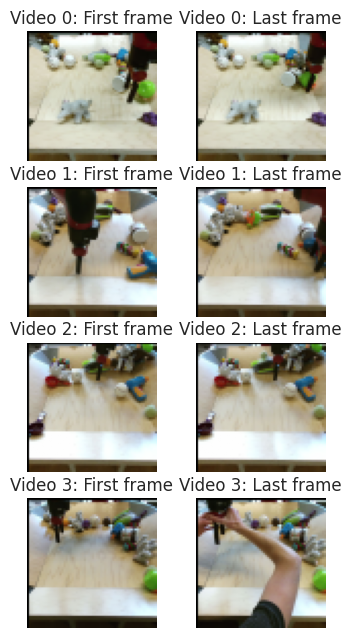### হাব মডিউল লোড করুন

hub_handle = 'https://tfhub.dev/google/tweening_conv3d_bair/1'

WARNING:tensorflow:Unable to create a python object for variable <tf.Variable 'video_discriminator/conv_0/weights:0' shape=(4, 4, 4, 3, 64) dtype=float32_ref> because it is a reference variable. It may not be visible to training APIs. If this is a problem, consider rebuilding the SavedModel after running tf.compat.v1.enable_resource_variables().

Warning:tensorflow:Unable to create a python object for variable <tf.Variable 'video_discriminator/conv_0/weights:0' shape=(4, 4, 4, 3, 64) dtype=float32_ref> because it is a reference variable. It may not be visible to training APIs. If this is a problem, consider rebuilding the SavedModel after running tf.compat.v1.enable_resource_variables().

Warning:tensorflow:Unable to create a python object for variable <tf.Variable 'video_discriminator/conv_0/LayerNorm/beta:0' shape=(64,) dtype=float32_ref> because it is a reference variable. It may not be visible to training APIs. If this is a problem, consider rebuilding the SavedModel after running tf.compat.v1.enable_resource_variables().

Warning:tensorflow:Unable to create a python object for variable <tf.Variable 'video_discriminator/conv_0/LayerNorm/beta:0' shape=(64,) dtype=float32_ref> because it is a reference variable. It may not be visible to training APIs. If this is a problem, consider rebuilding the SavedModel after running tf.compat.v1.enable_resource_variables().

Warning:tensorflow:Unable to create a python object for variable <tf.Variable 'video_discriminator/conv_0/LayerNorm/gamma:0' shape=(64,) dtype=float32_ref> because it is a reference variable. It may not be visible to training APIs. If this is a problem, consider rebuilding the SavedModel after running tf.compat.v1.enable_resource_variables().

Warning:tensorflow:Unable to create a python object for variable <tf.Variable 'video_discriminator/conv_0/LayerNorm/gamma:0' shape=(64,) dtype=float32_ref> because it is a reference variable. It may not be visible to training APIs. If this is a problem, consider rebuilding the SavedModel after running tf.compat.v1.enable_resource_variables().

Warning:tensorflow:Unable to create a python object for variable <tf.Variable 'video_discriminator/conv_1/weights:0' shape=(4, 4, 4, 64, 128) dtype=float32_ref> because it is a reference variable. It may not be visible to training APIs. If this is a problem, consider rebuilding the SavedModel after running tf.compat.v1.enable_resource_variables().

Warning:tensorflow:Unable to create a python object for variable <tf.Variable 'video_discriminator/conv_1/weights:0' shape=(4, 4, 4, 64, 128) dtype=float32_ref> because it is a reference variable. It may not be visible to training APIs. If this is a problem, consider rebuilding the SavedModel after running tf.compat.v1.enable_resource_variables().

Warning:tensorflow:Unable to create a python object for variable <tf.Variable 'video_discriminator/conv_1/LayerNorm/beta:0' shape=(128,) dtype=float32_ref> because it is a reference variable. It may not be visible to training APIs. If this is a problem, consider rebuilding the SavedModel after running tf.compat.v1.enable_resource_variables().

Warning:tensorflow:Unable to create a python object for variable <tf.Variable 'video_discriminator/conv_1/LayerNorm/beta:0' shape=(128,) dtype=float32_ref> because it is a reference variable. It may not be visible to training APIs. If this is a problem, consider rebuilding the SavedModel after running tf.compat.v1.enable_resource_variables().

Warning:tensorflow:Unable to create a python object for variable <tf.Variable 'video_discriminator/conv_0/weights:0' shape=(4, 4, 4, 3, 64) dtype=float32_ref> because it is a reference variable. It may not be visible to training APIs. If this is a problem, consider rebuilding the SavedModel after running tf.compat.v1.enable_resource_variables().

Warning:tensorflow:Unable to create a python object for variable <tf.Variable 'video_discriminator/conv_0/weights:0' shape=(4, 4, 4, 3, 64) dtype=float32_ref> because it is a reference variable. It may not be visible to training APIs. If this is a problem, consider rebuilding the SavedModel after running tf.compat.v1.enable_resource_variables().

Warning:tensorflow:Unable to create a python object for variable <tf.Variable 'video_discriminator/conv_0/LayerNorm/beta:0' shape=(64,) dtype=float32_ref> because it is a reference variable. It may not be visible to training APIs. If this is a problem, consider rebuilding the SavedModel after running tf.compat.v1.enable_resource_variables().

Warning:tensorflow:Unable to create a python object for variable <tf.Variable 'video_discriminator/conv_0/LayerNorm/beta:0' shape=(64,) dtype=float32_ref> because it is a reference variable. It may not be visible to training APIs. If this is a problem, consider rebuilding the SavedModel after running tf.compat.v1.enable_resource_variables().

Warning:tensorflow:Unable to create a python object for variable <tf.Variable 'video_discriminator/conv_0/LayerNorm/gamma:0' shape=(64,) dtype=float32_ref> because it is a reference variable. It may not be visible to training APIs. If this is a problem, consider rebuilding the SavedModel after running tf.compat.v1.enable_resource_variables().

Warning:tensorflow:Unable to create a python object for variable <tf.Variable 'video_discriminator/conv_0/LayerNorm/gamma:0' shape=(64,) dtype=float32_ref> because it is a reference variable. It may not be visible to training APIs. If this is a problem, consider rebuilding the SavedModel after running tf.compat.v1.enable_resource_variables().

Warning:tensorflow:Unable to create a python object for variable <tf.Variable 'video_discriminator/conv_1/weights:0' shape=(4, 4, 4, 64, 128) dtype=float32_ref> because it is a reference variable. It may not be visible to training APIs. If this is a problem, consider rebuilding the SavedModel after running tf.compat.v1.enable_resource_variables().

Warning:tensorflow:Unable to create a python object for variable <tf.Variable 'video_discriminator/conv_1/weights:0' shape=(4, 4, 4, 64, 128) dtype=float32_ref> because it is a reference variable. It may not be visible to training APIs. If this is a problem, consider rebuilding the SavedModel after running tf.compat.v1.enable_resource_variables().

Warning:tensorflow:Unable to create a python object for variable <tf.Variable 'video_discriminator/conv_1/LayerNorm/beta:0' shape=(128,) dtype=float32_ref> because it is a reference variable. It may not be visible to training APIs. If this is a problem, consider rebuilding the SavedModel after running tf.compat.v1.enable_resource_variables().

Warning:tensorflow:Unable to create a python object for variable <tf.Variable 'video_discriminator/conv_1/LayerNorm/beta:0' shape=(128,) dtype=float32_ref> because it is a reference variable. It may not be visible to training APIs. If this is a problem, consider rebuilding the SavedModel after running tf.compat.v1.enable_resource_variables().

Warning:tensorflow:Unable to create a python object for variable <tf.Variable 'video_discriminator/conv_0/weights:0' shape=(4, 4, 4, 3, 64) dtype=float32_ref> because it is a reference variable. It may not be visible to training APIs. If this is a problem, consider rebuilding the SavedModel after running tf.compat.v1.enable_resource_variables().

Warning:tensorflow:Unable to create a python object for variable <tf.Variable 'video_discriminator/conv_0/weights:0' shape=(4, 4, 4, 3, 64) dtype=float32_ref> because it is a reference variable. It may not be visible to training APIs. If this is a problem, consider rebuilding the SavedModel after running tf.compat.v1.enable_resource_variables().

Warning:tensorflow:Unable to create a python object for variable <tf.Variable 'video_discriminator/conv_0/LayerNorm/beta:0' shape=(64,) dtype=float32_ref> because it is a reference variable. It may not be visible to training APIs. If this is a problem, consider rebuilding the SavedModel after running tf.compat.v1.enable_resource_variables().

Warning:tensorflow:Unable to create a python object for variable <tf.Variable 'video_discriminator/conv_0/LayerNorm/beta:0' shape=(64,) dtype=float32_ref> because it is a reference variable. It may not be visible to training APIs. If this is a problem, consider rebuilding the SavedModel after running tf.compat.v1.enable_resource_variables().

Warning:tensorflow:Unable to create a python object for variable <tf.Variable 'video_discriminator/conv_0/LayerNorm/gamma:0' shape=(64,) dtype=float32_ref> because it is a reference variable. It may not be visible to training APIs. If this is a problem, consider rebuilding the SavedModel after running tf.compat.v1.enable_resource_variables().

Warning:tensorflow:Unable to create a python object for variable <tf.Variable 'video_discriminator/conv_0/LayerNorm/gamma:0' shape=(64,) dtype=float32_ref> because it is a reference variable. It may not be visible to training APIs. If this is a problem, consider rebuilding the SavedModel after running tf.compat.v1.enable_resource_variables().

Warning:tensorflow:Unable to create a python object for variable <tf.Variable 'video_discriminator/conv_1/weights:0' shape=(4, 4, 4, 64, 128) dtype=float32_ref> because it is a reference variable. It may not be visible to training APIs. If this is a problem, consider rebuilding the SavedModel after running tf.compat.v1.enable_resource_variables().

Warning:tensorflow:Unable to create a python object for variable <tf.Variable 'video_discriminator/conv_1/weights:0' shape=(4, 4, 4, 64, 128) dtype=float32_ref> because it is a reference variable. It may not be visible to training APIs. If this is a problem, consider rebuilding the SavedModel after running tf.compat.v1.enable_resource_variables().

Warning:tensorflow:Unable to create a python object for variable <tf.Variable 'video_discriminator/conv_1/LayerNorm/beta:0' shape=(128,) dtype=float32_ref> because it is a reference variable. It may not be visible to training APIs. If this is a problem, consider rebuilding the SavedModel after running tf.compat.v1.enable_resource_variables().

Warning:tensorflow:Unable to create a python object for variable <tf.Variable 'video_discriminator/conv_1/LayerNorm/beta:0' shape=(128,) dtype=float32_ref> because it is a reference variable. It may not be visible to training APIs. If this is a problem, consider rebuilding the SavedModel after running tf.compat.v1.enable_resource_variables().

Warning:tensorflow:Unable to create a python object for variable <tf.Variable 'video_discriminator/conv_0/weights:0' shape=(4, 4, 4, 3, 64) dtype=float32_ref> because it is a reference variable. It may not be visible to training APIs. If this is a problem, consider rebuilding the SavedModel after running tf.compat.v1.enable_resource_variables().

Warning:tensorflow:Unable to create a python object for variable <tf.Variable 'video_discriminator/conv_0/weights:0' shape=(4, 4, 4, 3, 64) dtype=float32_ref> because it is a reference variable. It may not be visible to training APIs. If this is a problem, consider rebuilding the SavedModel after running tf.compat.v1.enable_resource_variables().

Warning:tensorflow:Unable to create a python object for variable <tf.Variable 'video_discriminator/conv_0/LayerNorm/beta:0' shape=(64,) dtype=float32_ref> because it is a reference variable. It may not be visible to training APIs. If this is a problem, consider rebuilding the SavedModel after running tf.compat.v1.enable_resource_variables().

Warning:tensorflow:Unable to create a python object for variable <tf.Variable 'video_discriminator/conv_0/LayerNorm/beta:0' shape=(64,) dtype=float32_ref> because it is a reference variable. It may not be visible to training APIs. If this is a problem, consider rebuilding the SavedModel after running tf.compat.v1.enable_resource_variables().

Warning:tensorflow:Unable to create a python object for variable <tf.Variable 'video_discriminator/conv_0/LayerNorm/gamma:0' shape=(64,) dtype=float32_ref> because it is a reference variable. It may not be visible to training APIs. If this is a problem, consider rebuilding the SavedModel after running tf.compat.v1.enable_resource_variables().

Warning:tensorflow:Unable to create a python object for variable <tf.Variable 'video_discriminator/conv_0/LayerNorm/gamma:0' shape=(64,) dtype=float32_ref> because it is a reference variable. It may not be visible to training APIs. If this is a problem, consider rebuilding the SavedModel after running tf.compat.v1.enable_resource_variables().

Warning:tensorflow:Unable to create a python object for variable <tf.Variable 'video_discriminator/conv_1/weights:0' shape=(4, 4, 4, 64, 128) dtype=float32_ref> because it is a reference variable. It may not be visible to training APIs. If this is a problem, consider rebuilding the SavedModel after running tf.compat.v1.enable_resource_variables().

Warning:tensorflow:Unable to create a python object for variable <tf.Variable 'video_discriminator/conv_1/weights:0' shape=(4, 4, 4, 64, 128) dtype=float32_ref> because it is a reference variable. It may not be visible to training APIs. If this is a problem, consider rebuilding the SavedModel after running tf.compat.v1.enable_resource_variables().

Warning:tensorflow:Unable to create a python object for variable <tf.Variable 'video_discriminator/conv_1/LayerNorm/beta:0' shape=(128,) dtype=float32_ref> because it is a reference variable. It may not be visible to training APIs. If this is a problem, consider rebuilding the SavedModel after running tf.compat.v1.enable_resource_variables().

Warning:tensorflow:Unable to create a python object for variable <tf.Variable 'video_discriminator/conv_1/LayerNorm/beta:0' shape=(128,) dtype=float32_ref> because it is a reference variable. It may not be visible to training APIs. If this is a problem, consider rebuilding the SavedModel after running tf.compat.v1.enable_resource_variables().



### ভিডিওগুলি তৈরি এবং প্রদর্শন করুন

filled_frames = module(input_frames)['default'] / 255.0

# Show sequences of generated video frames.

# Concatenate start/end frames and the generated filled frames for the new videos.
generated_videos = np.concatenate([input_frames[:, :1] / 255.0, filled_frames, input_frames[:, 1:] / 255.0], axis=1)

for video_id in range(4):
fig = plt.figure(figsize=(10 * 2, 2))
for frame_id in range(1, 16):
ax = fig.add_axes([frame_id * 1 / 16., 0, (frame_id + 1) * 1 / 16., 1],
xmargin=0, ymargin=0)
ax.imshow(generated_videos[video_id, frame_id])
ax.axis('off')